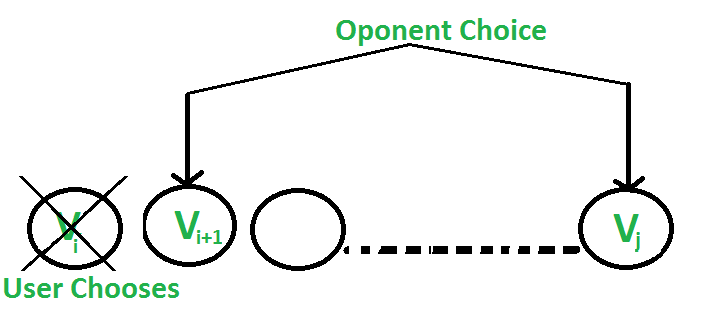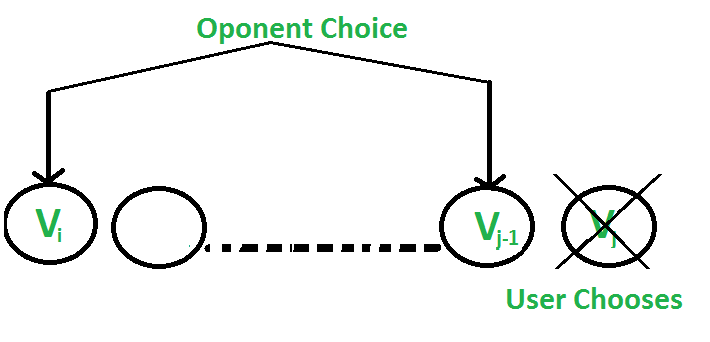Related Articles
Optimal Strategy for a Game | DP-31
• Difficulty Level : Hard
• Last Updated : 19 May, 2020

Consider a row of n coins of values v1 . . . vn, where n is even. We play a game against an opponent by alternating turns. In each turn, a player selects either the first or last coin from the row, removes it from the row permanently, and receives the value of the coin. Determine the maximum possible amount of money we can definitely win if we move first.

Note: The opponent is as clever as the user.

Let us understand the problem with few examples:

1. 5, 3, 7, 10 : The user collects maximum value as 15(10 + 5)
2. 8, 15, 3, 7 : The user collects maximum value as 22(7 + 15)

Does choosing the best at each move gives an optimal solution? No.
In the second example, this is how the game can be finished:

1. …….User chooses 8.
…….Opponent chooses 15.
…….User chooses 7.
…….Opponent chooses 3.
Total value collected by user is 15(8 + 7)
2. …….User chooses 7.
…….Opponent chooses 8.
…….User chooses 15.
…….Opponent chooses 3.
Total value collected by user is 22(7 + 15)

So if the user follows the second game state, the maximum value can be collected although the first move is not the best.

## Recommended: Please solve it on “PRACTICE” first, before moving on to the solution.

Approach: As both the players are equally strong, both will try to reduce the possibility of winning of each other. Now let’s see how the opponent can achieve this.

There are two choices:

1. The user chooses the ‘ith’ coin with value ‘Vi’: The opponent either chooses (i+1)th coin or jth coin. The opponent intends to choose the coin which leaves the user with minimum value.
i.e. The user can collect the value Vi + min(F(i+2, j), F(i+1, j-1) ).2. The user chooses the ‘jth’ coin with value ‘Vj’: The opponent either chooses ‘ith’ coin or ‘(j-1)th’ coin. The opponent intends to choose the coin which leaves the user with minimum value, i.e. the user can collect the value Vj + min(F(i+1, j-1), F(i, j-2) ).Following is the recursive solution that is based on the above two choices. We take a maximum of two choices.

```F(i, j) represents the maximum value the user
can collect from i'th coin to j'th coin.

F(i, j) = Max(Vi + min(F(i+2, j), F(i+1, j-1) ),
Vj + min(F(i+1, j-1), F(i, j-2) ))
As user wants to maximise the number of coins.

Base Cases
F(i, j) = Vi           If j == i
F(i, j) = max(Vi, Vj)  If j == i + 1
```

## C++

 `// C++ program to find out``// maximum value from a given``// sequence of coins``#include ``using` `namespace` `std;`` ` `// Returns optimal value possible``// that a player can collect from``// an array of coins of size n.``// Note than n must be even``int` `optimalStrategyOfGame(``    ``int``* arr, ``int` `n)``{``    ``// Create a table to store``    ``// solutions of subproblems``    ``int` `table[n][n];`` ` `    ``// Fill table using above``    ``// recursive formula. Note``    ``// that the table is filled``    ``// in diagonal fashion (similar``    ``// to http:// goo.gl/PQqoS),``    ``// from diagonal elements to``    ``// table[n-1] which is the result.``    ``for` `(``int` `gap = 0; gap < n; ++gap) {``        ``for` `(``int` `i = 0, j = gap; j < n; ++i, ++j) {``            ``// Here x is value of F(i+2, j),``            ``// y is F(i+1, j-1) and``            ``// z is F(i, j-2) in above recursive``            ``// formula``            ``int` `x = ((i + 2) <= j)``                        ``? table[i + 2][j]``                        ``: 0;``            ``int` `y = ((i + 1) <= (j - 1))``                        ``? table[i + 1][j - 1]``                        ``: 0;``            ``int` `z = (i <= (j - 2))``                        ``? table[i][j - 2]``                        ``: 0;`` ` `            ``table[i][j] = max(``                ``arr[i] + min(x, y),``                ``arr[j] + min(y, z));``        ``}``    ``}`` ` `    ``return` `table[n - 1];``}`` ` `// Driver program to test above function``int` `main()``{``    ``int` `arr1[] = { 8, 15, 3, 7 };``    ``int` `n = ``sizeof``(arr1) / ``sizeof``(arr1);``    ``printf``(``"%d\n"``,``           ``optimalStrategyOfGame(arr1, n));`` ` `    ``int` `arr2[] = { 2, 2, 2, 2 };``    ``n = ``sizeof``(arr2) / ``sizeof``(arr2);``    ``printf``(``"%d\n"``,``           ``optimalStrategyOfGame(arr2, n));`` ` `    ``int` `arr3[] = { 20, 30, 2, 2, 2, 10 };``    ``n = ``sizeof``(arr3) / ``sizeof``(arr3);``    ``printf``(``"%d\n"``,``           ``optimalStrategyOfGame(arr3, n));`` ` `    ``return` `0;``}`

## Java

 `// Java program to find out maximum``// value from a given sequence of coins``import` `java.io.*;`` ` `class` `GFG {`` ` `    ``// Returns optimal value possible``    ``// that a player can collect from``    ``// an array of coins of size n.``    ``// Note than n must be even``    ``static` `int` `optimalStrategyOfGame(``        ``int` `arr[], ``int` `n)``    ``{``        ``// Create a table to store``        ``// solutions of subproblems``        ``int` `table[][] = ``new` `int``[n][n];``        ``int` `gap, i, j, x, y, z;`` ` `        ``// Fill table using above recursive formula.``        ``// Note that the tableis filled in diagonal``        ``// fashion (similar to http:// goo.gl/PQqoS),``        ``// from diagonal elements to table[n-1]``        ``// which is the result.``        ``for` `(gap = ``0``; gap < n; ++gap) {``            ``for` `(i = ``0``, j = gap; j < n; ++i, ++j) {`` ` `                ``// Here x is value of F(i+2, j),``                ``// y is F(i+1, j-1) and z is``                ``// F(i, j-2) in above recursive formula``                ``x = ((i + ``2``) <= j)``                        ``? table[i + ``2``][j]``                        ``: ``0``;``                ``y = ((i + ``1``) <= (j - ``1``))``                        ``? table[i + ``1``][j - ``1``]``                        ``: ``0``;``                ``z = (i <= (j - ``2``))``                        ``? table[i][j - ``2``]``                        ``: ``0``;`` ` `                ``table[i][j] = Math.max(``                    ``arr[i] + Math.min(x, y),``                    ``arr[j] + Math.min(y, z));``            ``}``        ``}`` ` `        ``return` `table[``0``][n - ``1``];``    ``}`` ` `    ``// Driver program``    ``public` `static` `void` `main(String[] args)``    ``{``        ``int` `arr1[] = { ``8``, ``15``, ``3``, ``7` `};``        ``int` `n = arr1.length;``        ``System.out.println(``            ``""``            ``+ optimalStrategyOfGame(arr1, n));`` ` `        ``int` `arr2[] = { ``2``, ``2``, ``2``, ``2` `};``        ``n = arr2.length;``        ``System.out.println(``            ``""``            ``+ optimalStrategyOfGame(arr2, n));`` ` `        ``int` `arr3[] = { ``20``, ``30``, ``2``, ``2``, ``2``, ``10` `};``        ``n = arr3.length;``        ``System.out.println(``            ``""``            ``+ optimalStrategyOfGame(arr3, n));``    ``}``}`` ` `// This code is contributed by vt_m`

## Python3

 `# Python3 program to find out maximum ``# value from a given sequence of coins`` ` `# Returns optimal value possible that``# a player can collect from an array ``# of coins of size n. Note than n ``# must be even ``def` `optimalStrategyOfGame(arr, n):``     ` `    ``# Create a table to store ``    ``# solutions of subproblems ``    ``table ``=` `[[``0` `for` `i ``in` `range``(n)]``                ``for` `i ``in` `range``(n)]`` ` `    ``# Fill table using above recursive ``    ``# formula. Note that the table is ``    ``# filled in diagonal fashion ``    ``# (similar to http://goo.gl / PQqoS),``    ``# from diagonal elements to``    ``# table[n-1] which is the result. ``    ``for` `gap ``in` `range``(n):``        ``for` `j ``in` `range``(gap, n):``            ``i ``=` `j ``-` `gap``             ` `            ``# Here x is value of F(i + 2, j), ``            ``# y is F(i + 1, j-1) and z is ``            ``# F(i, j-2) in above recursive ``            ``# formula ``            ``x ``=` `0``            ``if``((i ``+` `2``) <``=` `j):``                ``x ``=` `table[i ``+` `2``][j]``            ``y ``=` `0``            ``if``((i ``+` `1``) <``=` `(j ``-` `1``)):``                ``y ``=` `table[i ``+` `1``][j ``-` `1``]``            ``z ``=` `0``            ``if``(i <``=` `(j ``-` `2``)):``                ``z ``=` `table[i][j ``-` `2``]``            ``table[i][j] ``=` `max``(arr[i] ``+` `min``(x, y),``                              ``arr[j] ``+` `min``(y, z))``    ``return` `table[``0``][n ``-` `1``]`` ` `# Driver Code``arr1 ``=` `[ ``8``, ``15``, ``3``, ``7` `]``n ``=` `len``(arr1)``print``(optimalStrategyOfGame(arr1, n))`` ` `arr2 ``=` `[ ``2``, ``2``, ``2``, ``2` `]``n ``=` `len``(arr2)``print``(optimalStrategyOfGame(arr2, n))`` ` `arr3 ``=` `[ ``20``, ``30``, ``2``, ``2``, ``2``, ``10``]``n ``=` `len``(arr3)``print``(optimalStrategyOfGame(arr3, n))`` ` `# This code is contibuted ``# by sahilshelangia`

## C#

 `// C# program to find out maximum``// value from a given sequence of coins``using` `System;`` ` `public` `class` `GFG {`` ` `    ``// Returns optimal value possible that a player``    ``// can collect from an array of coins of size n.``    ``// Note than n must be even``    ``static` `int` `optimalStrategyOfGame(``int``[] arr, ``int` `n)``    ``{``        ``// Create a table to store solutions of subproblems``        ``int``[, ] table = ``new` `int``[n, n];``        ``int` `gap, i, j, x, y, z;`` ` `        ``// Fill table using above recursive formula.``        ``// Note that the tableis filled in diagonal``        ``// fashion (similar to http:// goo.gl/PQqoS),``        ``// from diagonal elements to table[n-1]``        ``// which is the result.``        ``for` `(gap = 0; gap < n; ++gap) {``            ``for` `(i = 0, j = gap; j < n; ++i, ++j) {`` ` `                ``// Here x is value of F(i+2, j),``                ``// y is F(i+1, j-1) and z is``                ``// F(i, j-2) in above recursive formula``                ``x = ((i + 2) <= j) ? table[i + 2, j] : 0;``                ``y = ((i + 1) <= (j - 1)) ? table[i + 1, j - 1] : 0;``                ``z = (i <= (j - 2)) ? table[i, j - 2] : 0;`` ` `                ``table[i, j] = Math.Max(arr[i] + Math.Min(x, y),``                                       ``arr[j] + Math.Min(y, z));``            ``}``        ``}`` ` `        ``return` `table[0, n - 1];``    ``}`` ` `    ``// Driver program`` ` `    ``static` `public` `void` `Main()``    ``{``        ``int``[] arr1 = { 8, 15, 3, 7 };``        ``int` `n = arr1.Length;``        ``Console.WriteLine(``""` `+ optimalStrategyOfGame(arr1, n));`` ` `        ``int``[] arr2 = { 2, 2, 2, 2 };``        ``n = arr2.Length;``        ``Console.WriteLine(``""` `+ optimalStrategyOfGame(arr2, n));`` ` `        ``int``[] arr3 = { 20, 30, 2, 2, 2, 10 };``        ``n = arr3.Length;``        ``Console.WriteLine(``""` `+ optimalStrategyOfGame(arr3, n));``    ``}``}`` ` `// This code is contributed by ajit`

## PHP

 ``

Output:
```22
4
42
```

Complexity Analysis:

• Time Complexity: O(n2).
Use of a nested for loop brings the time complexity to n2.
• Auxiliary Space: O(n2).
As a 2-D table is used for storing states.

Note: The above solution can be optimized by using less number of comparisons for every choice. Please refer below.
Optimal Strategy for a Game | Set 2

Exercise:
Your thoughts on the strategy when the user wishes to only win instead of winning with the maximum value. Like the above problem, the number of coins is even.
Can the Greedy approach work quite well and give an optimal solution? Will your answer change if the number of coins is odd? Please see Coin game of two corners

This article is compiled by Aashish Barnwal. Please write comments if you find anything incorrect, or you want to share more information about the topic discussed above

Attention reader! Don’t stop learning now. Get hold of all the important DSA concepts with the DSA Self Paced Course at a student-friendly price and become industry ready.

My Personal Notes arrow_drop_up